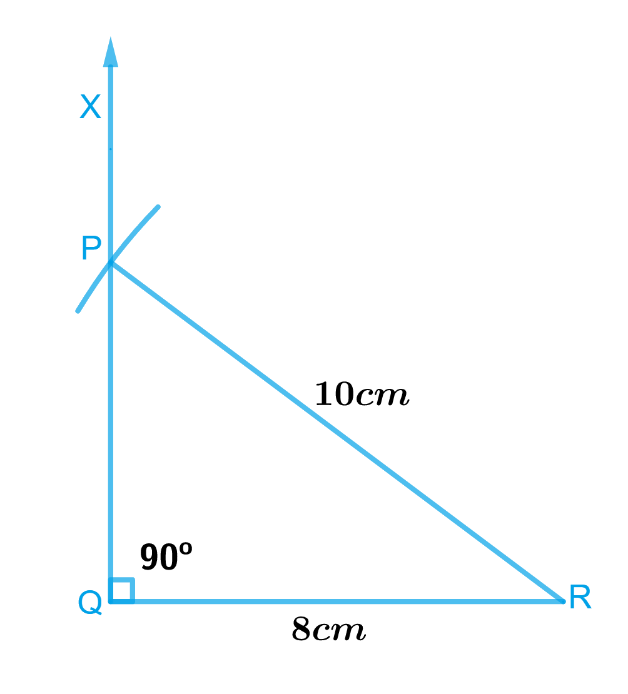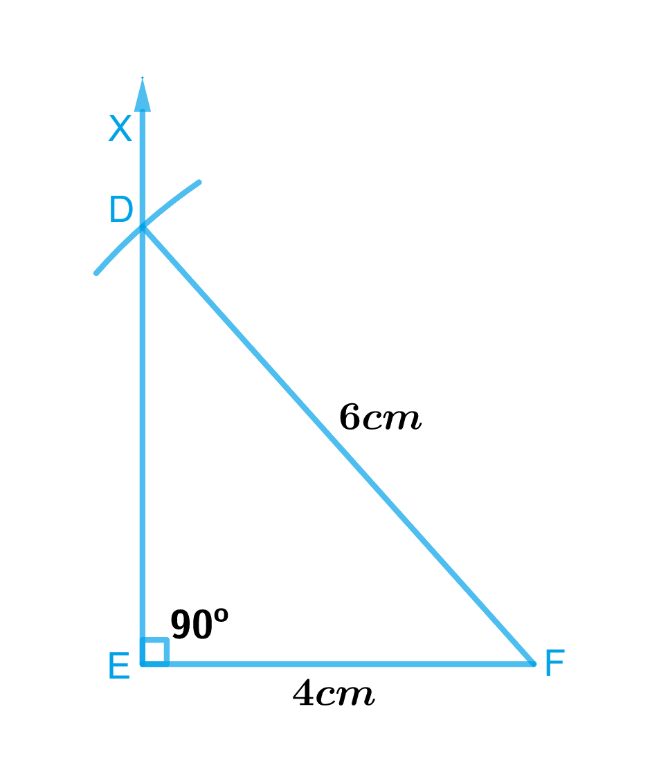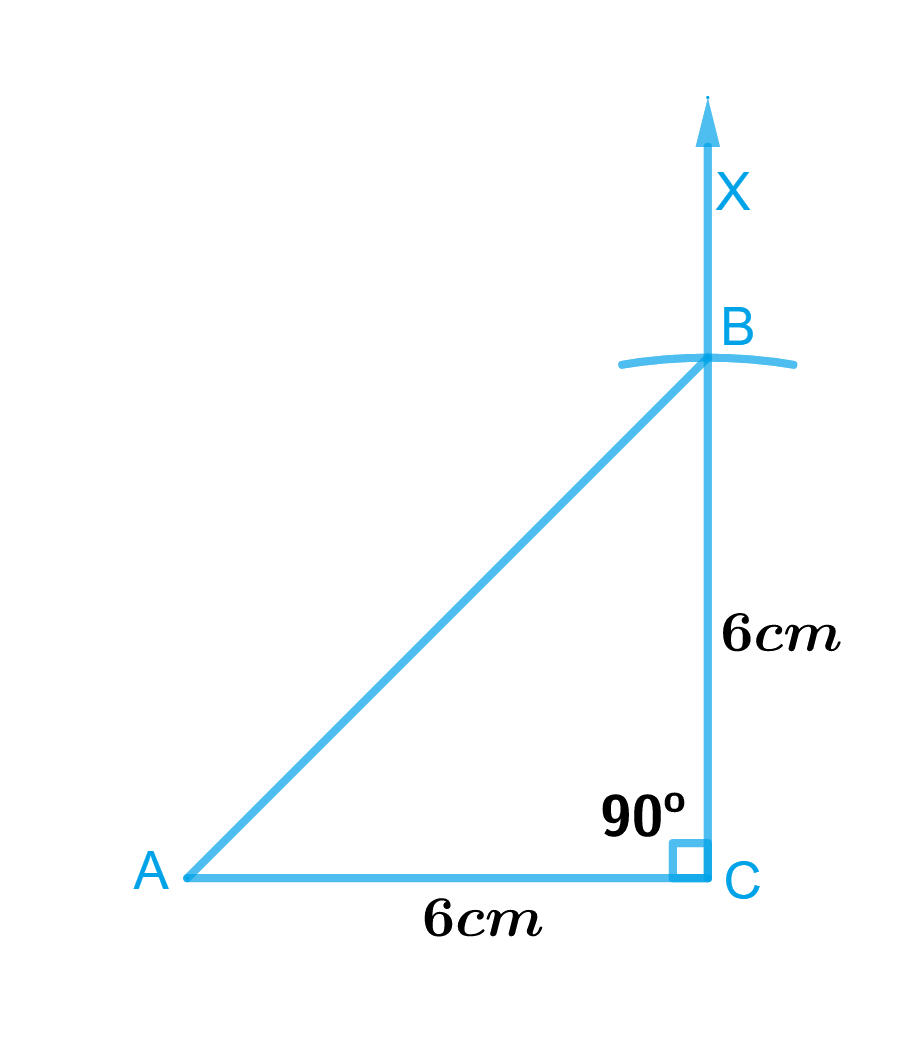# Exercise 10.5 Practical-Geometry -NCERT Solutions Class 7

## Chapter 10 Ex.10.5 Question 1

Construct the right angled $$ΔPQR$$, where $$m∠Q = 90^\circ$$, $$QR = 8 \rm{cm}$$ and $$PR = 10\, \rm{cm}.$$

### Solution

What is known?

Length of two side of a right angled $$ΔPQR$$.

To construct:

A right angled $$ΔPQR$$, where $$∠Q = 90^\circ$$, $$QR = 8 \rm{cm}$$ and $$PR = 10\, \rm{cm}.$$

Reasoning:

To construct a right-angled $$ΔPQR$$, where $$∠Q = 90^\circ$$, $$QR = 8\,\rm{cm}$$ and $$PR = 10 \,\rm{cm}$$, draw a rough sketch and mark the measures. Remember to mark the right angle and follow the steps given below.

Steps:Steps of construction :

1. Draw $$QR$$ of length $$8\, \rm{cm}.$$
2. At $$Q$$, draw $$QX\,\text{perpendicular}\,QR.$$
3. With $$R$$ as centre, draw an arc of radius $$10\,\rm{ cm}$$ which should intersect $$QX$$ at point $$P$$.
4. Join $$P$$ and $$R$$.

$$ΔPQR$$ is the required triangle.

## Chapter 10 Ex.10.5 Question 2

Construct a right-angled triangle whose hypotenuse is $$6\,\rm{ cm}$$ long and one of the legs is $$4\,\rm{ cm}$$ long.

### Solution

What is known?

Hypotenuse of a right-angled triangle and one of the legs.

To construct:

A right-angled triangle whose hypotenuse is $$6\,\rm{ cm}$$ long and one of the legs is $$4\,\rm{ cm}$$ long.

Reasoning:

To construct a right-angled triangle whose hypotenuse is $$6\,\rm{ cm}$$ long and one of the legs is $$4 \,\rm{cm}$$ long, draw a rough sketch and mark the measures. Remember to mark the right angle
and follow the steps given below.

Steps:Steps of construction

1. Draw a line segment $$EF$$ of length $$4\,\rm{ cm}.$$
2. At $$E$$, draw $$EX\,\text{perpendicular}\, EF.$$
3. With $$F$$ as centre, draw an arc of radius 6cm which should intersect $$EX$$ at point $$D.$$
4. Join $$D$$ with $$F$$.

$$ΔDEF$$ is the required triangle.

## Chapter 10 Ex.10.5 Question 3

Construct an isosceles right-angled triangle $$ABC$$, where $$m∠ACB = 90^\circ$$ and $$AC = 6\,\rm{ cm}.$$

### Solution

To construct:

An isosceles right-angled triangle $$ABC$$, where $$m∠ACB = 90^\circ$$ and $$AC = 6\,\rm{ cm}.$$

What is known?

Length of side of an isosceles right-angled triangle $$ABC$$,$$AC = 6\,\rm{cm}$$ and $$∠ACB = 90^\circ$$.

Reasoning:

Since $$ΔABC$$ is an isosceles right-angled triangle, where $$∠ACB = 90^\circ$$ and $$AC = 6\,\rm{ cm}$$. Therefore, length of the other equal side, we can take is $$BC = 6\,\rm{cm}$$. To construct this triangle, follow the steps given below.

Steps:Steps of construction

1. Draw a line segment $$CA$$ of length $$6\,\rm{cm}.$$
2. At point $$C,$$ draw $$CX$$ perpendicular $$CA.$$
3. With $$C$$ as centre, draw an arc of radius $$6\,\rm{cm}$$ which should intersect $$CX$$ at point $$B.$$
4. Join $$A$$ and $$B.$$

Triangle $$ABC$$ is the required isosceles right-angles triangle.

Instant doubt clearing with Cuemath Advanced Math Program